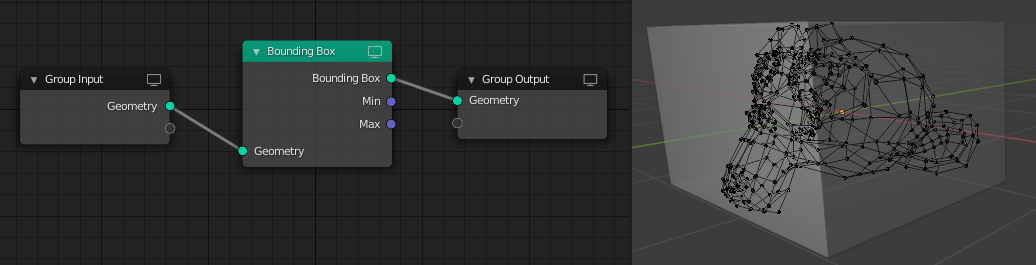# Bounding Box Node

The Bounding Box node creates a box with the minimum volume that encapsulates the geometry of the input. The node also can output the vector positions of the bounding dimensions.

For instanced geometry, a bounding box is computed for each instance rather than the whole geometry. To compute the bounding box of the whole geometry, you must use a Realize Instances Node.

## Входы

Геометрия

Standard geometry input.

## Свойства

Этот узел не содержит свойств.

## Выходы

Bounding Box (Ограничительная рамка)

The resulting box that encapsulate the input geometry.

Мин.

The coordinates corresponding to the box’s -X, -Y, -Z position values, i.e. how far the box extends in each of the negative axes directions.

Макс.

The coordinates corresponding to the box’s +X, +Y, +Z position values, i.e. how far the box extends in each of the positive axes directions.

## ПримерBounding Box node used to create a box that encapsulates the geometry of the monkey mesh.